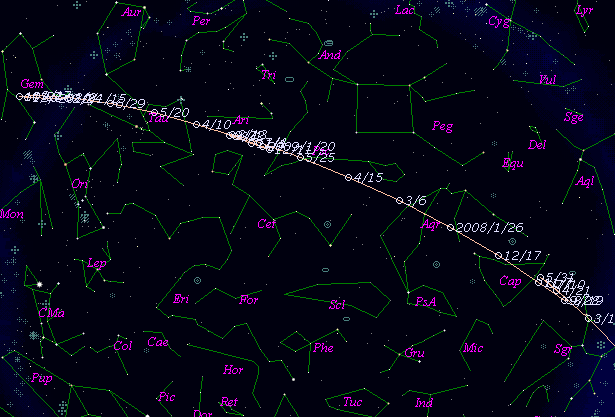# \$B%(%k%9%H(B-\$B%T%5%mWB@1(B

133P/(7968) Elst-Pizarro (2007)###\$B50F;MWAG(B

```Perturbed ephemeris below is based on elements from CCO 14.

Epoch = 2454280.50000 TT   T = 2454280.85938 TT
Peri. =  132.06733         a =     3.1583849 AU
Node  =  160.18540 J2000.0 e =     0.1635761
Incl. =    1.38614         q =     2.6417487 AU
```

###\$B@1?^(B###\$B8wEYJQ2=(B

```        H = 15.5  G = 0.15
```##### \$B50F;MWAG\$O(BMinor Planet & Comet Ephemeris Service\$B\$G7W;;\$7\$?\$b\$N\$G\$9!#(B \$B@1?^\$O(B StellaNavigator Ver.2.0 for Windows (\$B%"%9%H%m%"!<%D(B \$BJTCx(B / \$B%"%9%-!<=PHG6I4)(B) \$B\$G:n@.\$7\$?\$b\$N\$G\$9!#(B \$B8wEY%0%i%U\$O(BComet for Windows\$B\$G:n@.\$7\$?\$b\$N\$G\$9!#(B